Courses

# Ionic Equilibrium Introduction (Part-1) Chemistry Notes | EduRev

## Chemistry : Ionic Equilibrium Introduction (Part-1) Chemistry Notes | EduRev

The document Ionic Equilibrium Introduction (Part-1) Chemistry Notes | EduRev is a part of the Chemistry Course Physical Chemistry.
All you need of Chemistry at this link: Chemistry

1. Conductors: Substances which show conductivity or flow of current are called conductors.

(a) Metallic conductor: These substances show conductivit y due to motion of free electrons.

Ex.: Graphite

(b) Ionic Conductor: These substances show conductivity due to movement of free ions.

Ionic conductors are further classified into two types:

(i) Strong Electrolytes: Are those whose ionization is almost 100% in aqueous solut ion.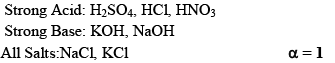(ii) Weak Electrolytes: Whose degree of dissociation is < 10-15%. Generally covalent compounds are weak electrolytes e.g. NH4OH, CH3COOH.

Strong and Weak electrolytes is a value term its only depends upon degree of ionization. Sometimes covalent compounds acts as a strong electrolytes in highly diluted solution.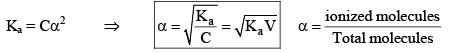“The fraction of total number of molecules of electrolytes dissolved, that ionizes at equilibrium is called degree of ionization or degree of dissociation.”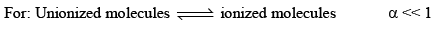Generally strong and weak electrolyte property depends upon nature of solvent NaCl in H2O acts strong electrolyte whereas CH3COOH is weak electrolyte in water. But in liquid HN3. The dissociation of NaCl and CH3COOH both are same.

2. Acids Bases and Salts

A. Arrhenius Concept:

Arrhenius Acid: according to Arrhenius concept, an acid is a substance that dissociates to given hydrogen ions when dissolved in water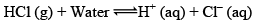The symbol aq indicates that the ions are hydrated i.e., associated with one or more water Molecules.

Arrhenius Base: According to Arrhenius concept, a base is a substance that dissociated into Hydroxy / ions when dissolved in water.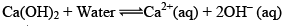The greater the number of H+ or OH ions given by an acid or a base in water, the greater is the strength of the acid or the base.

B. Proton Transfer Theory:

Concept of Lowry and Bronsted According to their concept – “An acid is defined as substance which has a tendency to donate a proton to any other substance and a base is a substance which has a tendency to accept a proton from any other substance”

In other words, Acid is a proton donor and a base is proton acceptor.

Conjugate Acids and Bases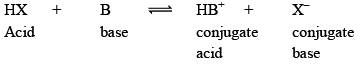The limitation of the Bronsted Lowry Theory is that the extent to which a dissolved substance can act as an acid or a base depends largely on solvent.

C. Lewis Concept:

An acid is a molecule / ion which can accept an electron pain wit h the formative of a coordinated bond.

Ex: BF3, AlCl3

A base is any Molecule which has a lone pair of electrons which can be donated.

Ex: NH3, PH3, OH–

Few acid: base reactions are: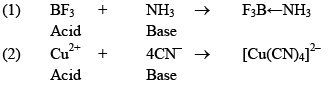3. Degree of Dissociation (α)

As the name suggests, α tells us the extent of dissociat ion. When an electrolyte is dissolved in a solvent H2O, it spontaneously dissociates into ions.

The degree of dissociation ( α) of an electrolyte is the fraction of one mole of the electrolyte that has dissociated under the given conditions.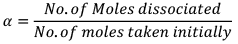Dissociation of weak acid: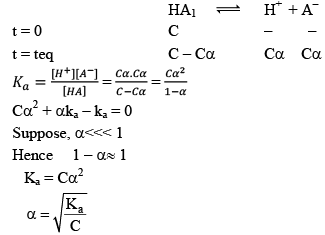This expression is obtained after neglection when α< 0.05. Otherwise, solve quadratic equation to find the value of α.

4. Factors affecting the value of degree of dissociation

1) Ostwald’s Dilution Law: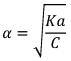As dilution increases, concentration decreases which ultimately leads to increase in degree of dissociation.

2) Temperature

We know, dissociation is endothermic Reaction. ΔH = +ve. Hence, when temperature increases then Keq increases.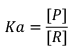As Keq increases, conc.Of product increases. Ultimately degree of dissociation (Δα) increases:

5. Ostwald’s Dilution Law

Ostwald pointed out that like chemical equilibrium, in ionic equilibrium we can apply law of mass action. An equilibrium between ionized and unionized molecules.

Consider a binary electrolyte having conc. C and degree of dissociation is α.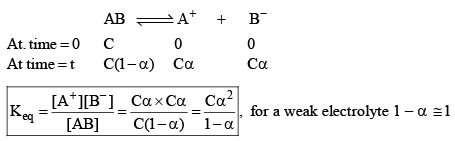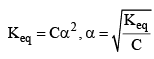If 1 mole of AB is present in ‘V’ litre of solution.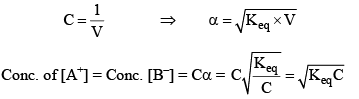Limitation

(i) This is only for weak electrolytes not for strong electrolytes.

(ii) This law is not applicable for strong electrolytes because strong electrolytes are almost completely ionized at all dilution hence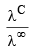does not give accurate results.

6. Relative strength of Acids and Bases

For weak acids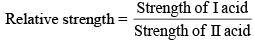For acids HA1 if concentration is C1 and degree of dissociat ions α1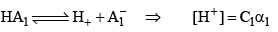For HA2 = if concentration is C2 and degree of dissociat ion is α2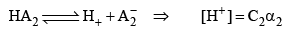Then, relative strength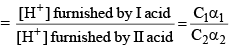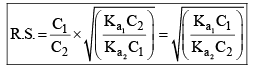If C1 and C2 are same, then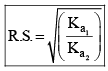7. Common ion Effect

The degree of dissociation of a weak electrolyte is suppressed by the addition of another electrolyte the common ion. Common ion = weak + strong acid/base.

Quantitative Aspect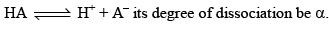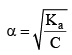BA (another electrolyte) added whose molarity is M, due to his the new degree of dissociation becomes α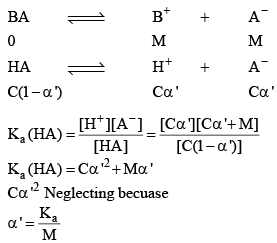As a result of common ion effect, the conc. of the ion, which is not common changes to make the Ka constant, because K only depends upon temperature not on concentration.

Note: The use of this phenomenon is made in quantities analysis to adjust the conc. of S – ions in second group (reagent H2S and HCl) and OH– ion conc. in third group (NH4Cl + NH4OH).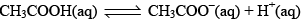The degree of ionization of this weak acid can be calculated by the approximately relation: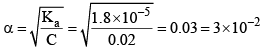Now let us calculate the degree of ionization when the solution also contains 0.01 M sodium acetate.Sodium acetate being a strong electrolyte would be completely io nized in solut ion. Let x mo l L–1 of acetic acid be ionized.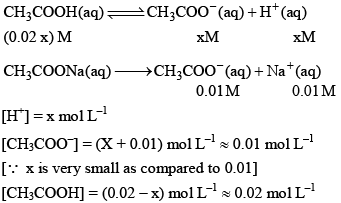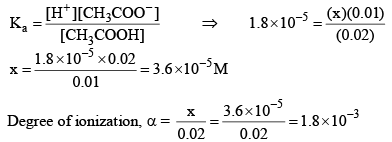Thus, it may noted that the degree of ionization of acetic acid has decreased fro m 3 × 10–2 to 1.8 × 10–3 due to the presence of sodium acetate.

α = 1.5 × 10–8

8. Ionization of Water

Water is a weak electrolyte. It does not dissociate completely, the undissociated water and the dissociated H+, OH remain the equilibrium. Let us take pure water whose density is 1 gram/c.c. and hence its concentration would be (1000/18 = 55.55 M). Let the degree of dissociat ion of water be α.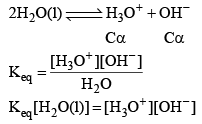Since conc. of pure water remain constant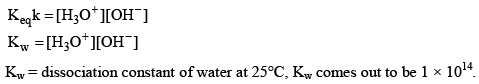Kw depends upon temperature dissociation of water gives equal no. of H3O+ and OH ions.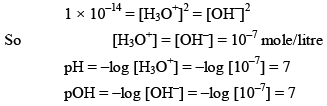Note: In case of water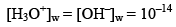But in case of acidic or basic solution.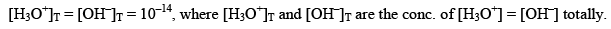Effect of temperature on Ionic product of water

As mentioned earlier, with increase in temperature value of equilibrium constant increases.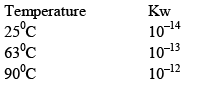9. pH Calculation

The pH of a solution is the negative logarithm (to the base 10) of the concentration (in moles per litre) of hydrogen ions which it conations.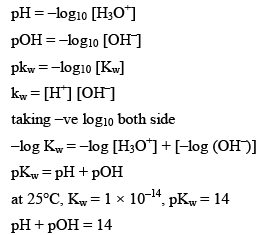Kw = is also called as auto protolysis constant, it increases with temperature. Since wit h increase in temperature association of water increases. Therefore the value o f Kw increases as the temperature is increased, however at all temperature [H3O+] remain equal to [OH] in pure water.

10.  pH determination for Weak Acids

Weak acids do not dissociate completely in the water their % degree of dissociation is very less. E.g. lets takes CH3COOH (C mole/litre and having degree dissociation a).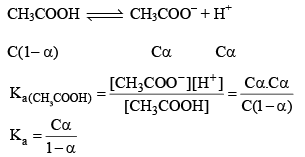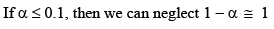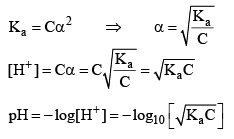Note: For calculat ing the pH of a weak acid first of all we calculate α from the equation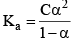if αcomes out to be ≤ 0.1 then 1 – α will be taken as one and we use formula Ka = Cα2 otherwise we would be use the formula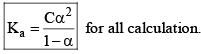Determination of pH of acids or bases

For strong acid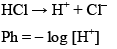If conc. of HCl is less than 10–6 M than we take conc. of H+ form water into accounts i.e. 10–7. Otherwise we would neglect the conc. of H+ from water.

e.g. find the pH of 10–8 M HCl

According to rule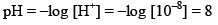But pH of an acid can’t be 8 so we have to take contribution of H+ from water

Total H+ ion in solution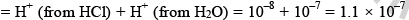mole/litre and hence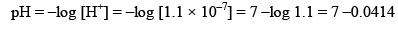pH = 6.9586 (Acidic).

11. pH for the mixture of Weak Acid and Strong Acid

Let strong acid be HB whose conc. is C1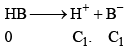And weak acid whose conc. is C2 and degree of dissociation is α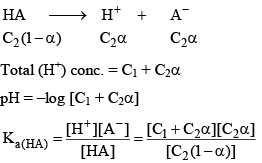12. pH calculation of solution of a mixture of two weak Acids in water

Let two weak acids be HA and HB and their conc. are C1 and C2, α1 is the degree of dissociat ion of HA in presence of HB (due to common ion defect) and α2 be degree of dissociation of HB in presence of HA. IN aqueous solution of HA and HB following equilibrium exists.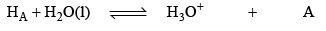conc. at eqi.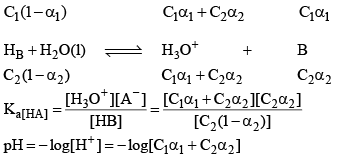13. pH of a dibasic Acids and Polyprotic Acid

Let’s take the eg. of a dibasic acid H2A. Assuming both dissociation is weak. Let the initial conc. of H2A is C and α1 and α2 be degree of dissociation for first and second dissociation.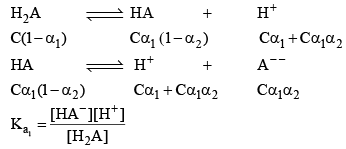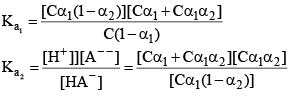After solving for α1 and α2. We can calculate the H+ conc.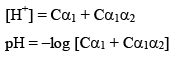14. pH of mixture

Let one litre of acidic solution of pH2 be mixed with two litre of other acidic solution of pH3. The resultant pH of mixture can be evaluated in the following way.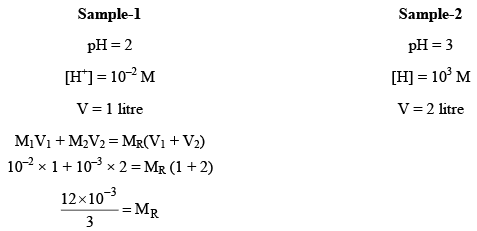4 × 10–3 = MR (Here, MR = Resultant molarity)

pHR = –log [4 × 10–3] = 2.3980

15. Buffer Solution

A Buffer solution is that which resist the pH change by addition of small quantity of acid or alkali.

There are three types of buffer (i) Acidic Buffer (ii) Basic Buffer (iii) Salt Buffer

Mechanism of Buffer Action:

Let us see why CH3COOH is a Buffer while NaCl is not. CH3COOH4 exists almost entirely in form of it’s io n CH3COO or NH4+ if an acid is added to that solut ion, the H+ ion furnished by the acid combine with CH3COO ions to form feebly dissociated molecule of CH3COOH.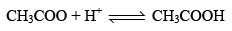Since most of the H+ ions added are taken up by CH3COO to form CH3COOH which itself slightly dissociated, the pH of CH3COONH4 changes only slightly.

Now, suppose a base is added to CH3COONH4 solution the OH furnished by the base will be taken up by NH4+ ion to form feebly dissociated NH4OH.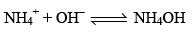Since most of the OH ions are taken up by NH4+ ions to form feebly dissociated NH4OH. Due to this very little change in the pH of CH3COONH4 solution occurs.

Now let us see why a solution of NaCl is not a Buffer. In aqueous solution it is almost entirely dissociated into Na+ and Cl. If H+ ions are added to this solution the H+ combines with Cl to form HCl which completely dissociated due to strong electrolyte hence pH falls.

If OH ions are added to the solution, it will combine wit h Na+ to form NaOH which will almost completely dissociated. Hence pH will rise.

16. Acid Buffer

A very common acidic buffer is prepared by mixing equimolar solutions of acetic acid and sodium acetate. Acetic acid is very slightly dissociated while sodium acetate, being a salt is almost completely dissociated. The mixture thus contains CH3COOH molecules as well as CH3COO and Na+ ions. Let us consider the buffer action of this mixture.

Suppose a strong acid is added to the above mixture. The H+ ions added will be taken up immediately by CH3COO ions to form very slightly dissociated CH3COOH: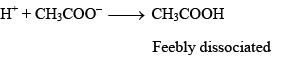Thus, the H+ ions added are neutralized by the acetate ions present in the mixture. There is very little change in the pH of the mixture.

If, on the other hand, a strong base is added, the OH ions added are neutralized by the acetic acid present in the mixture.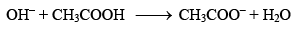Thus, again, there is very little change in the pH of the mixture.

Offer running on EduRev: Apply code STAYHOME200 to get INR 200 off on our premium plan EduRev Infinity!

## Physical Chemistry

77 videos|83 docs|32 tests

,

,

,

,

,

,

,

,

,

,

,

,

,

,

,

,

,

,

,

,

,

;